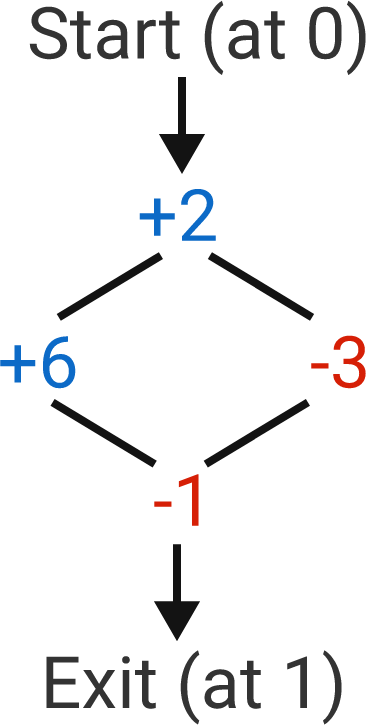# Number Maze

Logic Level 2

Starting at "Start" with a value $x$ at 0, and then travelling among the maze adding and subtracting to $x$ as specified, is it possible to leave the maze with $x$ at 1?It is possible to cycle back and forth between two rooms, but the value of 1 must be had at the exit, not just midway through the path.

For example, one possible path from Start (which doesn't succeed) would be to +2 $(x = 2),$ -3 $(x = -1),$ +2 $(x = 1),$ + 6 $(x = 7),$ -1 $(x = 6).$

×